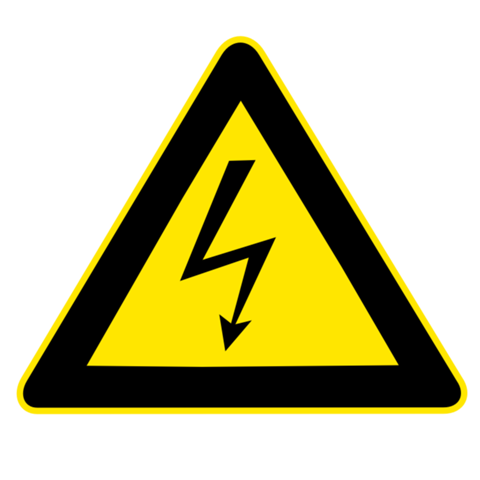# Difference between volts and wattsVolts and watts are two of the most common and key terms in the field of Electrical and Electronics Engineering. Most of the times these terms confuse the newbie or those who have just started learning Electrical sciences.

Even though these two terms sounds alike, they are different from each other. Here is a simple explanation for that.

## Volts

“Volt” is the unit by which voltage or potential difference between any two points is measured. Simply, “volt” is the unit of voltage. It is denoted by ‘V’. One volt is equal to the potential difference developed when one joule of work is done in moving one coulomb of charge from one point to another. It is measured using voltmeter.

## Watts

Watt is the unit of power. One watt is equal to the electric power generated when one joule of work is done for one second.Power = Voltage x Current and its unit is Watts. Electric power is measured using wattmeter.

Hence watts is the measure of electric power whereas volt is the measure of electrical potential difference between two point and are entirely different from each other.

### Related Searches:

#### Watts vs volts Difference between volts and watts vape Difference between volts and watts e cig Difference between a watt and sunlight The difference between volts and watts

Sharing is caring